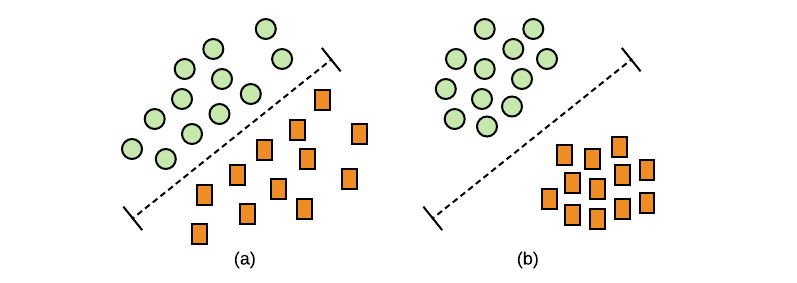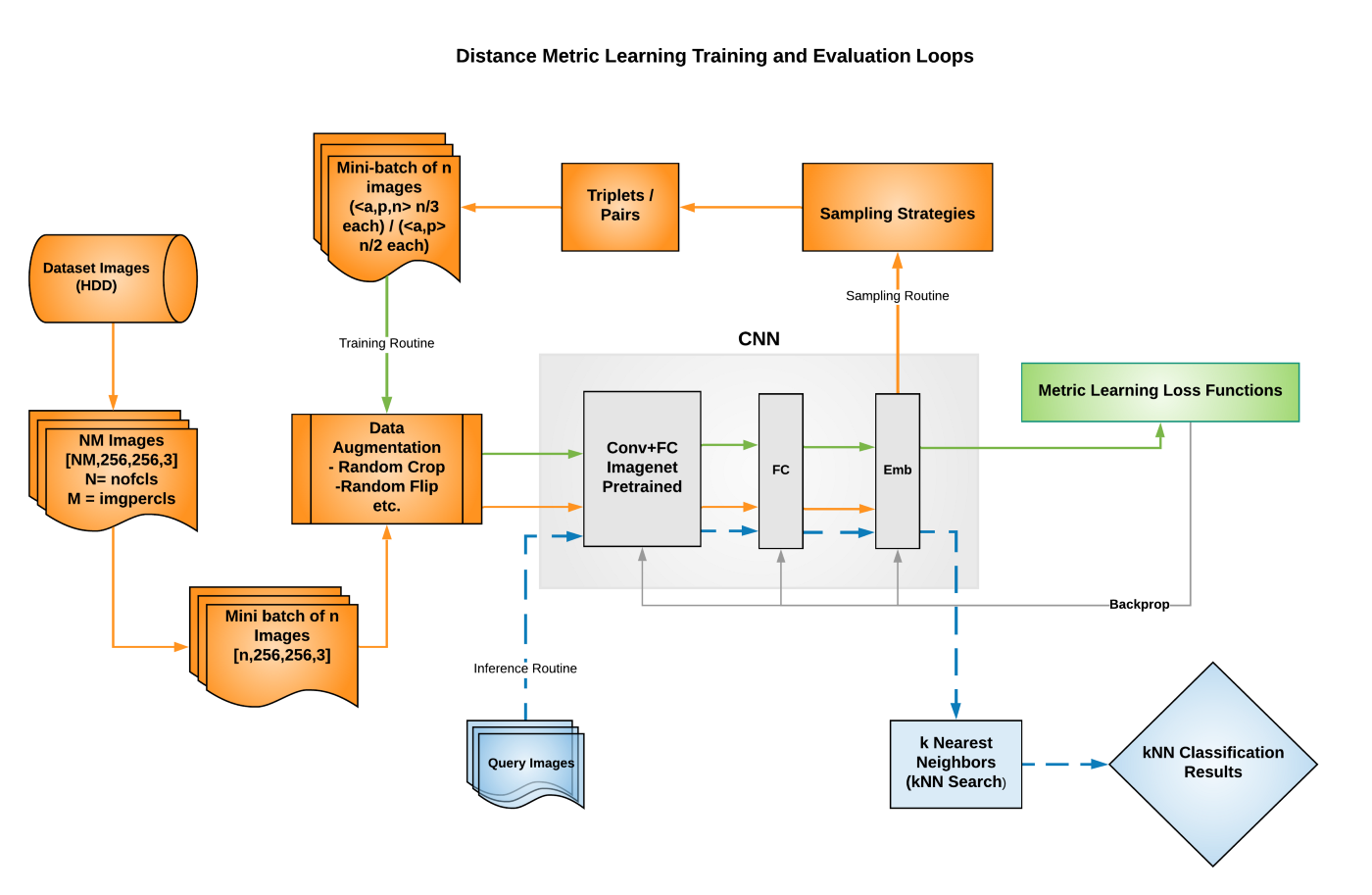## 1. Contrastive Loss(对比损失)

$$L = \frac{1}{m} \sum _{(i,j)} ^{m/2} y_{i,j} D_{i,j}^2 + (1 - y_{i,j})[\alpha - D_{i, j}^2]^+$$

$D_{i,j} = ||f(x_i) - f(x_j)||^2$ 为图片$x_i$ 和 $x_j$ 的特征 $f(x_i)$ 和 $f(x_j)$ 之间的距离.

$y_{i,j} = +/-1$ 为指示器，如果 $(x_i, x_j)$ 是相似标签，则值为1，否则值为0.

$[.]^+$ 是 hinge 损失函数 $max(0, .)$.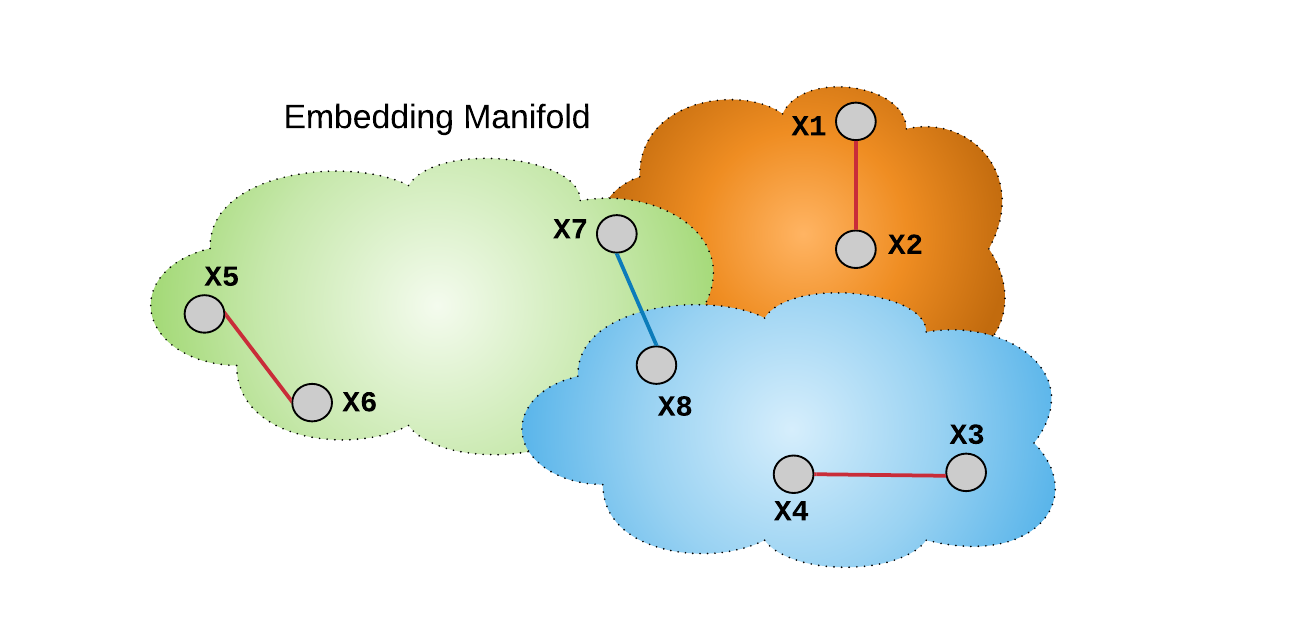- 首先，随机选择 16 款产品；

 - 然后，从16款产品的每一款随机选择 2 张图片；

 - 则，在 mini-batch B=32时，生成得到了 16 个正样本对，15*16 个负样本对.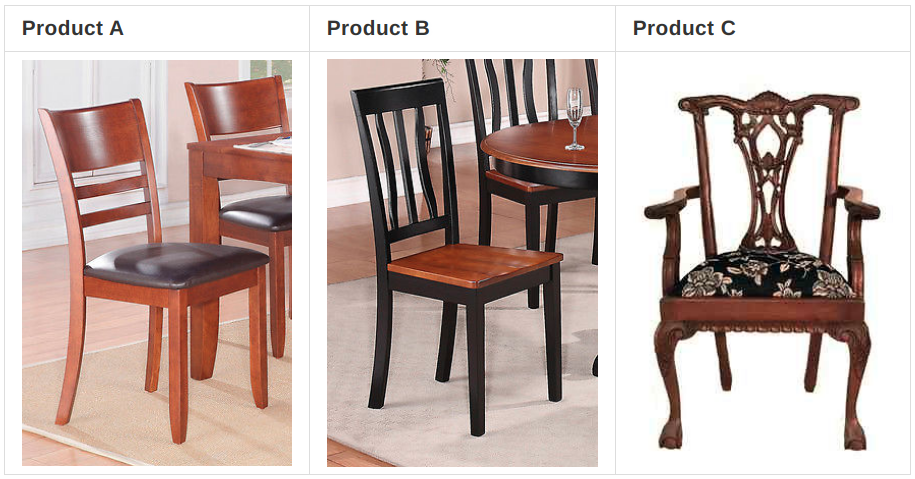## 2. Triplet Loss(三元损失)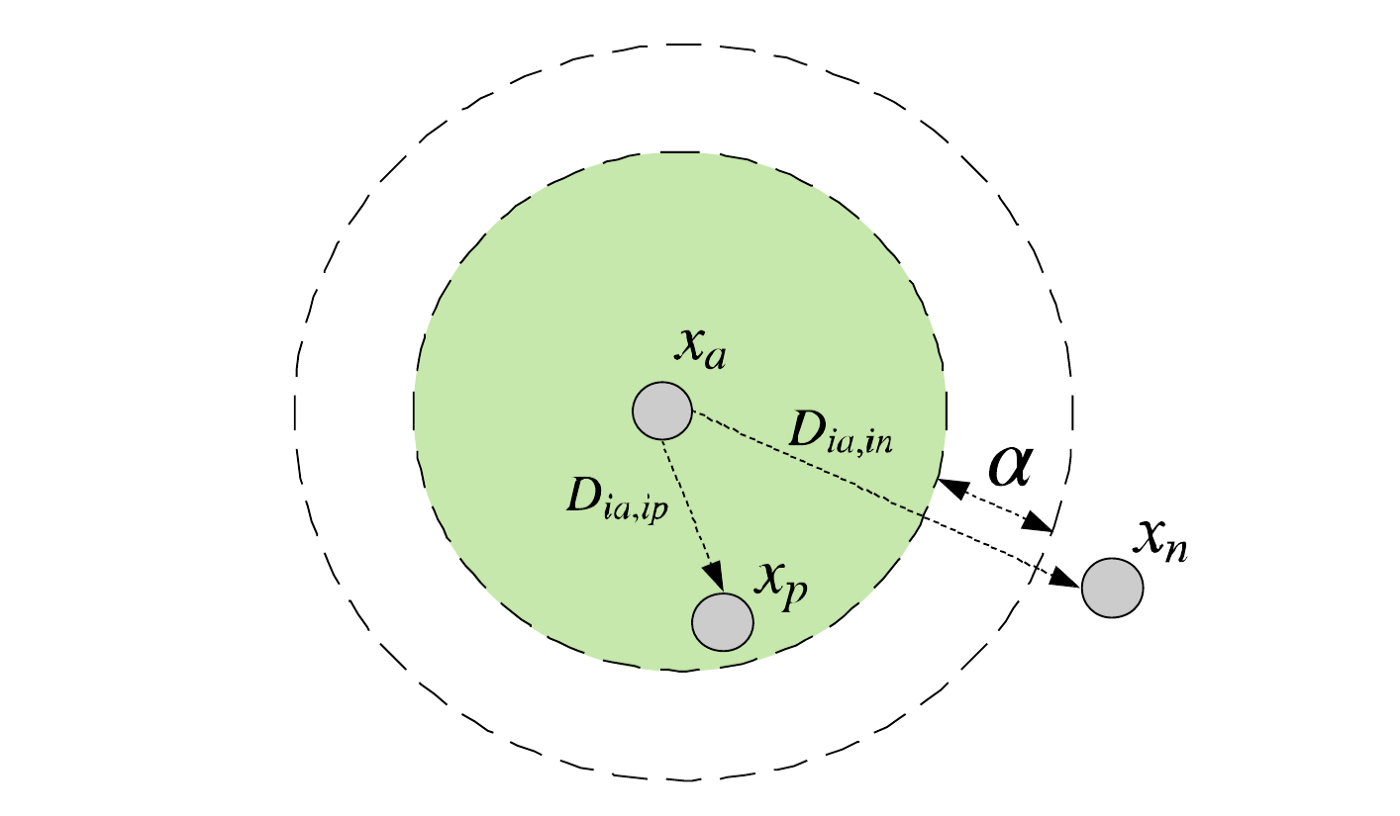$$L = \frac{3}{2m} \sum_{i}^{m/3} [D_{ia, ip}^2 - D_{ia, in}^2 + \alpha]_+$$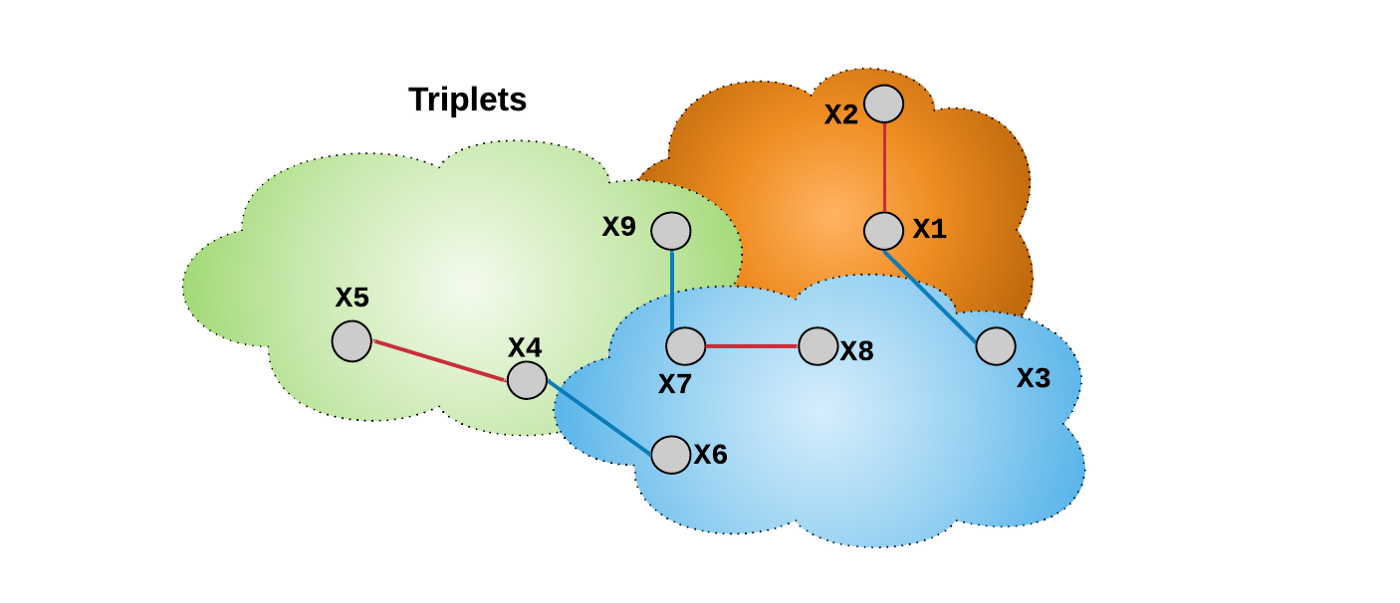## 3. Lifted Structure Loss

Lifted structure loss 的思想是，采用所有的 O(B^2) 样本对，而不是 O(B) 个独立的样本对，来提升 mini-batch 的优化. 如图：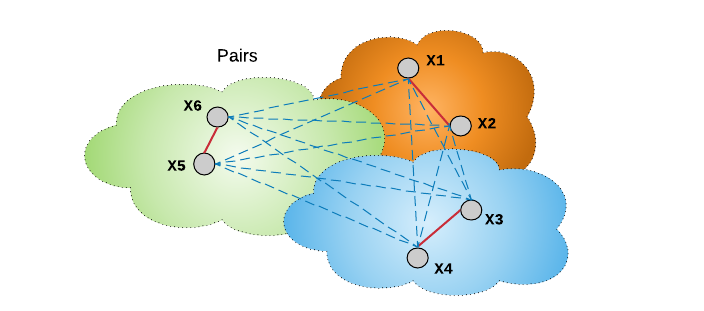Lifted structure loss 中，样本对的节点是分别与 mini-batch 内所有负样本节点进行连接的. 与三元损失函数不同之处是，其不需要通过预定义 anchor 来定义负样本，而忽略了 mini-batch 内所有的其他可用的负样本. Lifted structure loss 将每个正样本看作是一个 anchor，并寻找其负样本，以计算损失函数. 其有助于网络训练的速度更快，收敛性更好.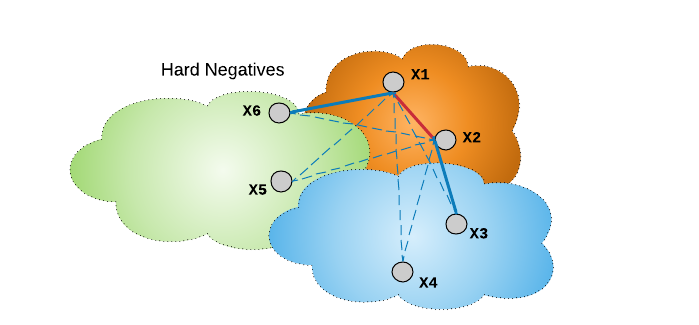Lifted struceture loss 函数最小化平滑上界，以稳定网络训练. 具体来说，其定义如：

$$L_{i,j} = log(\sum_{(i, k) \in N} e^{(\alpha - D_{i,k})} + \sum_{(i, l) \in N}e^{(\alpha - D_{j, l})} ) + D_{i,j}$$

$$L = \frac{1}{2|P|} \sum_{(i, j) \in P} max(0, L_{i,j})^2$$

$P$ 和 $N$ 分别表示 mini-batch 内所有的正样本对和负样本对.

## 4. N-Pair Loss

Multi-class N-pair loss 类似于 Lifted Structure loss，其在计算 mini-batch 的损失函数值时利用了多个负样本，且并不像三元损失和对比损失一样收敛速度变慢. 如图.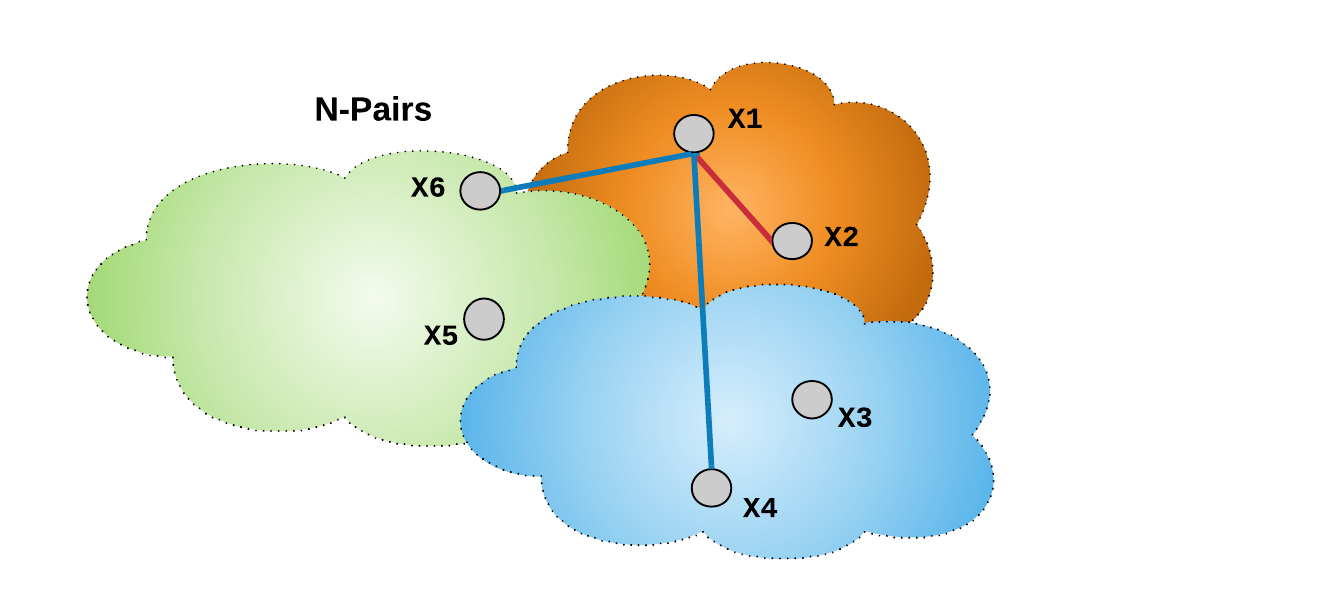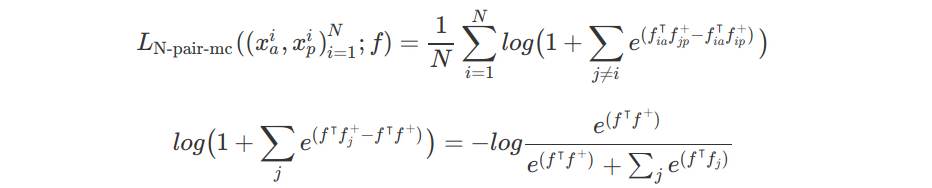N-pair loss 相比于 Lifted Structure loss 的一个优势在于，其尝试以概率方式计算正样本对和负样本对的余弦(cos)相似性. 换句话说，N-pair loss 计算一对特征之间的余弦相似性，采用 mini-batch 的成对比较，提升属于相同标签的特征的概率. 由于余弦相似性度量(即概率) 具有尺度不变形，如下图，N-pair loss 在训练时具有对特征变化的鲁棒性.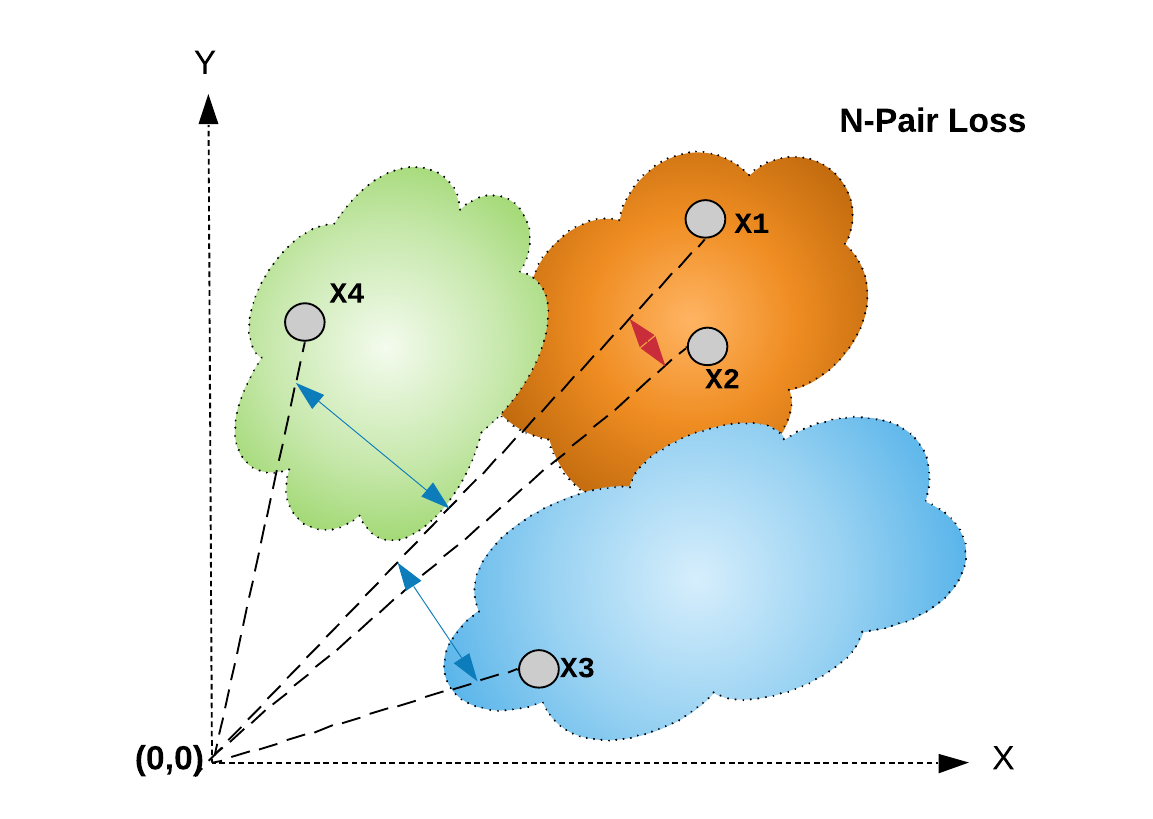## 5. Angular LossAngular loss 的几个有点在于：

 - 不同于欧式距离，角度距离(余弦距离) 是平移不变相似性度量. 当考虑几何角度时，不仅受益于尺度不变性，还引入了旋转不变性. 即使在训练时，图像特征尺度变化比较频繁，对于固定的 margin $\alpha$，$\ang n \le \alpha$ 往往是满足的. 简而言之，损失函数中的几何角度视角对于特征图的局部变化更加鲁棒.

 - 余弦规则解释了 $\ang n$ 的计算需要三元组的所有三个边. 而原来的三元组仅考虑了两个边. 新增的约束有利于优化的鲁棒性和有效性.

 - 当采用欧式距离时，对于损失函数来说，损失margin m 的选择不是一项直观的任务. 主要是因为，随着数据集大小的增长，目标标签的类内变化会比较大. 该超参数的调参至关重要. 相比而言，由于其尺度不变性，设置 $\alpha$ 就简单很多.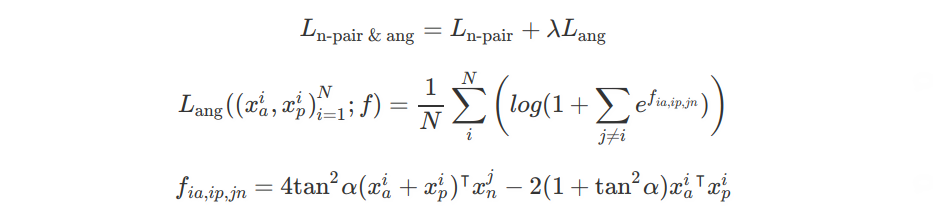## 6. Divergence Loss

Divergence loss 是一个正则项，可以将其添加到度量学习损失函数，以联合监督. 其增加不同组合模块学习到的图像特征之间的距离. 换句话说，其鼓励每个模块去学习输入图像的不同属性，公式如，

$$L(x_i, y_i) = \sum_m L_{1, (m)} (x_i, y_i) + \lambda L_2(x_i)$$

$$L_2(x) = \sum_i \sum_{a, p} [m - D_{ia, ip}^2]_+$$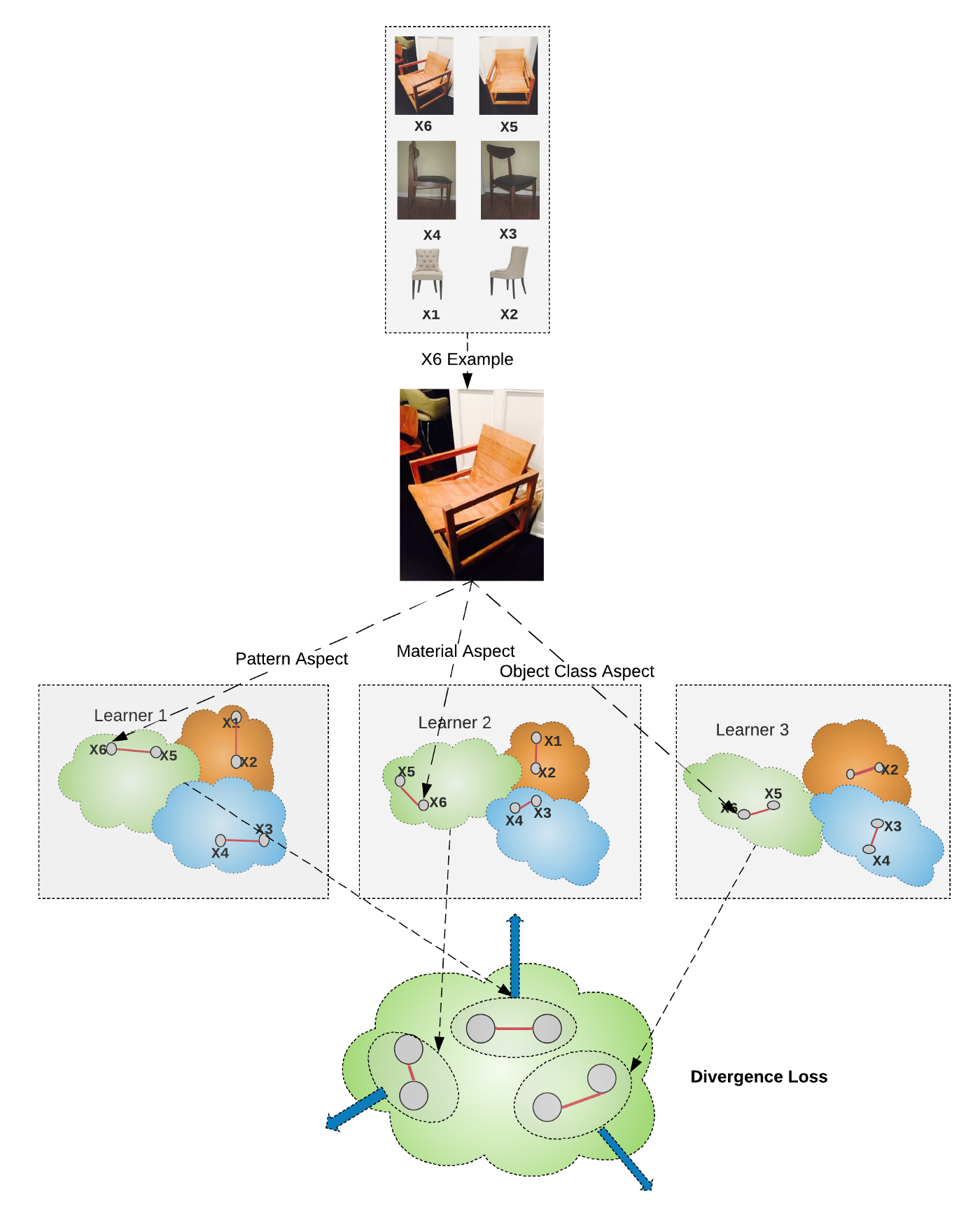## 7. 总结

SGD 计算时，通过随机采样数据集和适当的 batchsize 来构建 minibatch. 然而， 例如，如果在三元损失函数中，采用随机采样来构建 mini-batches，很有可能在最后没有任何正样本对或负样本对，导致其梯度非常小. 因此，在 mini-batch 梯度处理中，虽然可以通过 mini-batch 降低计算量，但是如果 mini-batches 的损失贡献小，则有可能需要更多计算量，以达到最优的点. 因此，训练数据集的采样和minibatch的构建策略，使其能够对损失函数包含充分的信息，而又不要太大，对于加快度量学习网络的训练至关重要.

## 参考

 Hadsell, R., Chopra, S., & LeCun, Y. (2006, June). Dimensionality reduction by learning an invariant mapping. In 2006 IEEE Computer Society Conference on Computer Vision and Pattern Recognition (CVPR’06) (Vol. 2, pp. 1735–1742). IEEE.

 Wen, Y., Zhang, K., Li, Z., & Qiao, Y. (2016, October). A discriminative feature learning approach for deep face recognition. In European conference on computer vision (pp. 499–515). Springer, Cham.

 Schroff, F., Kalenichenko, D., & Philbin, J. (2015). Facenet: A unified embedding for face recognition and clustering. In Proceedings of the IEEE conference on computer vision and pattern recognition (pp. 815–823).

 Oh Song, H., Xiang, Y., Jegelka, S., & Savarese, S. (2016). Deep metric learning via lifted structured feature embedding. In Proceedings of the IEEE Conference on Computer Vision and Pattern Recognition (pp. 4004–4012).

 Sohn, K. (2016). Improved deep metric learning with multi-class n-pair loss objective. In Advances in Neural Information Processing Systems (pp. 1857–1865).

 Wang, J., Zhou, F., Wen, S., Liu, X., & Lin, Y. (2017). Deep metric learning with angular loss. In Proceedings of the IEEE International Conference on Computer Vision (pp. 2593–2601).

 Kim, W., Goyal, B., Chawla, K., Lee, J., & Kwon, K. (2018). Attention-based ensemble for deep metric learning. In Proceedings of the European Conference on Computer Vision (ECCV) (pp. 736–751).

Last modification：April 12th, 2021 at 01:44 pm